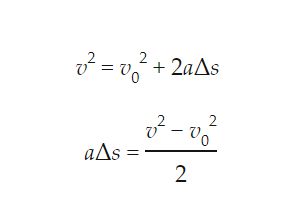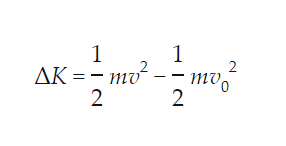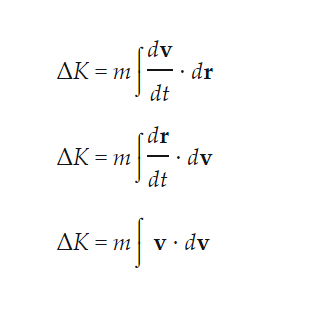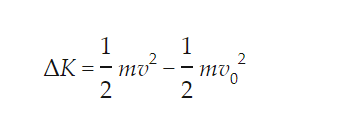# Derivation Of Kinetic EnergyThe kinetic energy of a body is the energy that is possessed due to its motion. Kinetic energy is the work needed to accelerate an object of a given mass from rest to its stated velocity. The derivation of kinetic energy is one of the most common questions asked in the examination. To excel in their examinations, students must properly understand the kinetic energy derivation method.

Kinetic energy depends upon the body’s velocity and mass. If the body’s velocity is zero, then the kinetic energy will also be zero. The derivation of kinetic energy is given below so that students can understand the concept more effectively. The kinetic energy formula derivation can be done using algebra and calculus. Both methods are explained below.

## Derivation of Kinetic Energy using Algebra

The kinetic energy derivation using only algebra is one of the best ways to understand the formula in-depth.

Starting with the work-energy theorem and then adding Newton’s second law of motion, we can say that,Now, taking the kinematics equation and rearranging it, we getCombining the 2 expressions we get,Now we already know that kinetic energy is the energy that it possessed due to its motion. So the kinetic energy at rest should be zero. Therefore, we can say that kinetic energy is:## Derivation of Kinetic Energy using Calculus

The derivation of kinetic energy using calculus is given below. To derive an expression for kinetic energy using calculus, we will not need to assume anything about the acceleration.

Starting with the work-energy theorem and Newton’s second law of motion we can say thatNow rearranging the differential terms to get the function and the integral into an agreement.Now, we know that the kinetic energy of a body at rest is zero. So we can say that the kinetic energy is:## Frequently Asked Questions – FAQs

### What is the definition of kinetic energy?

The kinetic energy is the measure of the work an object can do by virtue of its motion.

### Can the kinetic energy be negative?

The kinetic energy cannot be negative because the square of the speed gives a non-negative number, and the mass cannot be negative.

### When is the kinetic energy maximum?

The kinetic energy is maximum when the potential energy is maximum, and this occurs when the velocity is maximum and the mass is at the equilibrium position.

### What happens to the kinetic energy when the speed decreases?

When the speed decreases, the kinetic energy will be transformed into potential energy, thermal energy or other forms of energy.

### How is the kinetic energy different from the potential energy?

The energy possessed by the body by virtue of its position is known as potential energy. The energy possessed by the body by virtue of its motion is known as kinetic energy.

Stay tuned with BYJU’S and learn various other derivations of Physics formulas.

Test your knowledge on Kinetic energy derivation